# Setup for 2 Player a Game of Food Chain Magnate

Originally generated on 10/11/2018 8:13:33 PM
A direct link to this setup is https://www.BoardgameHelpers.com/FoodChainMagnate/LoadGame.aspx?id=\$2a\$04\$YzZVeRFV9KiM4tqXxZC0Pe

## Player Setup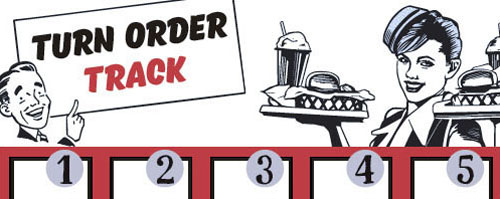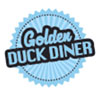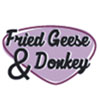## Other Setup

Bank starts with \$100.00
Remove these Billboards: #12, #15, #16
Number of 1x Employee cards used = 1

## Map (Broken Roads)

Click here to view Map Tile Lettering Key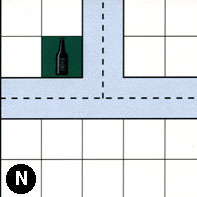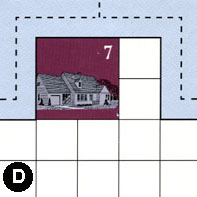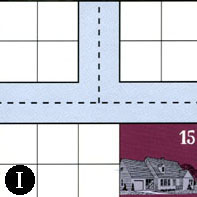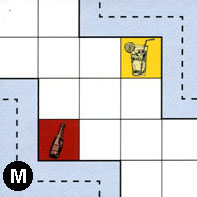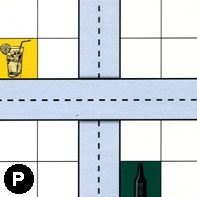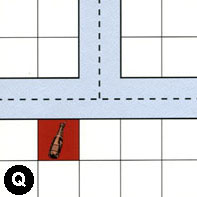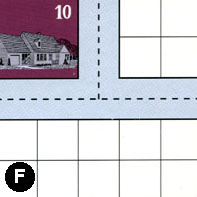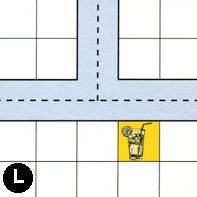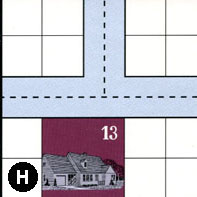### Map Stats

Map Option = Broken Roads
Total Number of Tiles = 9
Total Number of Starting Houses = 4
Total Number of Beer Spots = 2
Total Number of Soda Spots = 2
Total Number of Lemonade Spots = 3
Total Number of Drink Spots = 7
Number of independent path systems = 6
Number of independent neighborhoods = 8

Path System 1
Size = Medium
Number of Tiles = 1
Number of Paths = 3
Contains Loop(s) = No
Number of Starting Houses = 1
Starting Houses Pct of Map (%) = 25
Number of Beer Spots = 1
Number of Soda Spots = 0
Number of Lemonade Spots = 0
Number of Drink Spots = 1
Drink Spots Pct of Map (%) = 14
Tile Ids = N
Paths = NWN, NWE, NNE What do these mean?
Starting House Ids = 0
Path System 2
Size = Medium
Number of Tiles = 2
Number of Paths = 4
Contains Loop(s) = No
Number of Starting Houses = 2
Starting Houses Pct of Map (%) = 50
Number of Beer Spots = 1
Number of Soda Spots = 0
Number of Lemonade Spots = 0
Number of Drink Spots = 1
Drink Spots Pct of Map (%) = 14
Tile Ids = D, P
Paths = DWN, DNE, PNS, DWE What do these mean?
Starting House Ids = 0, 7
Path System 3
Size = Medium
Number of Tiles = 2
Number of Paths = 6
Contains Loop(s) = No
Number of Starting Houses = 3
Starting Houses Pct of Map (%) = 75
Number of Beer Spots = 0
Number of Soda Spots = 1
Number of Lemonade Spots = 0
Number of Drink Spots = 1
Drink Spots Pct of Map (%) = 14
Tile Ids = I, Q
Paths = IWN, INE, QWN, QNE, QWE, IWE What do these mean?
Starting House Ids = 0, 13, 15
Path System 4
Size = Medium
Number of Tiles = 2
Number of Paths = 4
Contains Loop(s) = No
Number of Starting Houses = 2
Starting Houses Pct of Map (%) = 50
Number of Beer Spots = 0
Number of Soda Spots = 1
Number of Lemonade Spots = 0
Number of Drink Spots = 1
Drink Spots Pct of Map (%) = 14
Tile Ids = F, M
Paths = MWS, FWE, FWN, FNE What do these mean?
Starting House Ids = 0, 10
Path System 5
Size = Medium
Number of Tiles = 2
Number of Paths = 6
Contains Loop(s) = No
Number of Starting Houses = 2
Starting Houses Pct of Map (%) = 50
Number of Beer Spots = 0
Number of Soda Spots = 0
Number of Lemonade Spots = 1
Number of Drink Spots = 1
Drink Spots Pct of Map (%) = 14
Tile Ids = H, L
Paths = LWN, HWE, HWN, HNE, LWE, LNE What do these mean?
Starting House Ids = 0, 13
Path System 6
Size = Small
Number of Tiles = 2
Number of Paths = 2
Contains Loop(s) = No
Number of Starting Houses = 1
Starting Houses Pct of Map (%) = 25
Number of Beer Spots = 0
Number of Soda Spots = 0
Number of Lemonade Spots = 2
Number of Drink Spots = 2
Drink Spots Pct of Map (%) = 29
Tile Ids = M, P
Paths = MEN, PWE What do these mean?
Starting House Ids = 0

Neighborhood 1
Total Size = Small
Number of Total Spaces = 4
Number of Empty Spaces (for new houses & gardens) = 3
Number of Beer Spots = 1
Number of Soda Spots = 0
Number of Lemonade Spots = 0
Number of Drink Spots = 1
Number of Starting Houses = 0
Starting Houses Pct of Map (%) = 0
Tile Ids = N
Neighborhood 2
Total Size = Huge
Number of Total Spaces = 119
Number of Empty Spaces (for new houses & gardens) = 101
Number of Beer Spots = 1
Number of Soda Spots = 2
Number of Lemonade Spots = 3
Number of Drink Spots = 6
Number of Starting Houses = 3
Starting Houses Pct of Map (%) = 75
Tile Ids = D, F, H, I, L, M, N, P, Q
Starting House Ids = 7, 13, 15
Neighborhood 3
Total Size = Small
Number of Total Spaces = 4
Number of Empty Spaces (for new houses & gardens) = 4
Number of Beer Spots = 0
Number of Soda Spots = 0
Number of Lemonade Spots = 0
Number of Drink Spots = 0
Number of Starting Houses = 0
Starting Houses Pct of Map (%) = 0
Tile Ids = I
Neighborhood 4
Total Size = Medium
Number of Total Spaces = 8
Number of Empty Spaces (for new houses & gardens) = 8
Number of Beer Spots = 0
Number of Soda Spots = 0
Number of Lemonade Spots = 0
Number of Drink Spots = 0
Number of Starting Houses = 0
Starting Houses Pct of Map (%) = 0
Tile Ids = I, Q
Neighborhood 5
Total Size = Small
Number of Total Spaces = 4
Number of Empty Spaces (for new houses & gardens) = 4
Number of Beer Spots = 0
Number of Soda Spots = 0
Number of Lemonade Spots = 0
Number of Drink Spots = 0
Number of Starting Houses = 0
Starting Houses Pct of Map (%) = 0
Tile Ids = F
Neighborhood 6
Total Size = Small
Number of Total Spaces = 4
Number of Empty Spaces (for new houses & gardens) = 0
Number of Beer Spots = 0
Number of Soda Spots = 0
Number of Lemonade Spots = 0
Number of Drink Spots = 0
Number of Starting Houses = 1
Starting Houses Pct of Map (%) = 25
Tile Ids = F
Starting House Ids = 10
Neighborhood 7
Total Size = Medium
Number of Total Spaces = 8
Number of Empty Spaces (for new houses & gardens) = 8
Number of Beer Spots = 0
Number of Soda Spots = 0
Number of Lemonade Spots = 0
Number of Drink Spots = 0
Number of Starting Houses = 0
Starting Houses Pct of Map (%) = 0
Tile Ids = H, L
Neighborhood 8
Total Size = Small
Number of Total Spaces = 4
Number of Empty Spaces (for new houses & gardens) = 4
Number of Beer Spots = 0
Number of Soda Spots = 0
Number of Lemonade Spots = 0
Number of Drink Spots = 0
Number of Starting Houses = 0
Starting Houses Pct of Map (%) = 0
Tile Ids = H

v2.1.5